# Precalculus : Find a Vector Equation When Given Two Points

## Example Questions

### Example Question #1 : Find A Vector Equation When Given Two Points

What is the vector that connects the point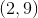to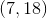?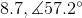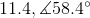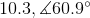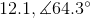Explanation:

The first step when solving for a vetor is to find the length of the vector. It can be helpful to visualize the system as a right triangle as seen below: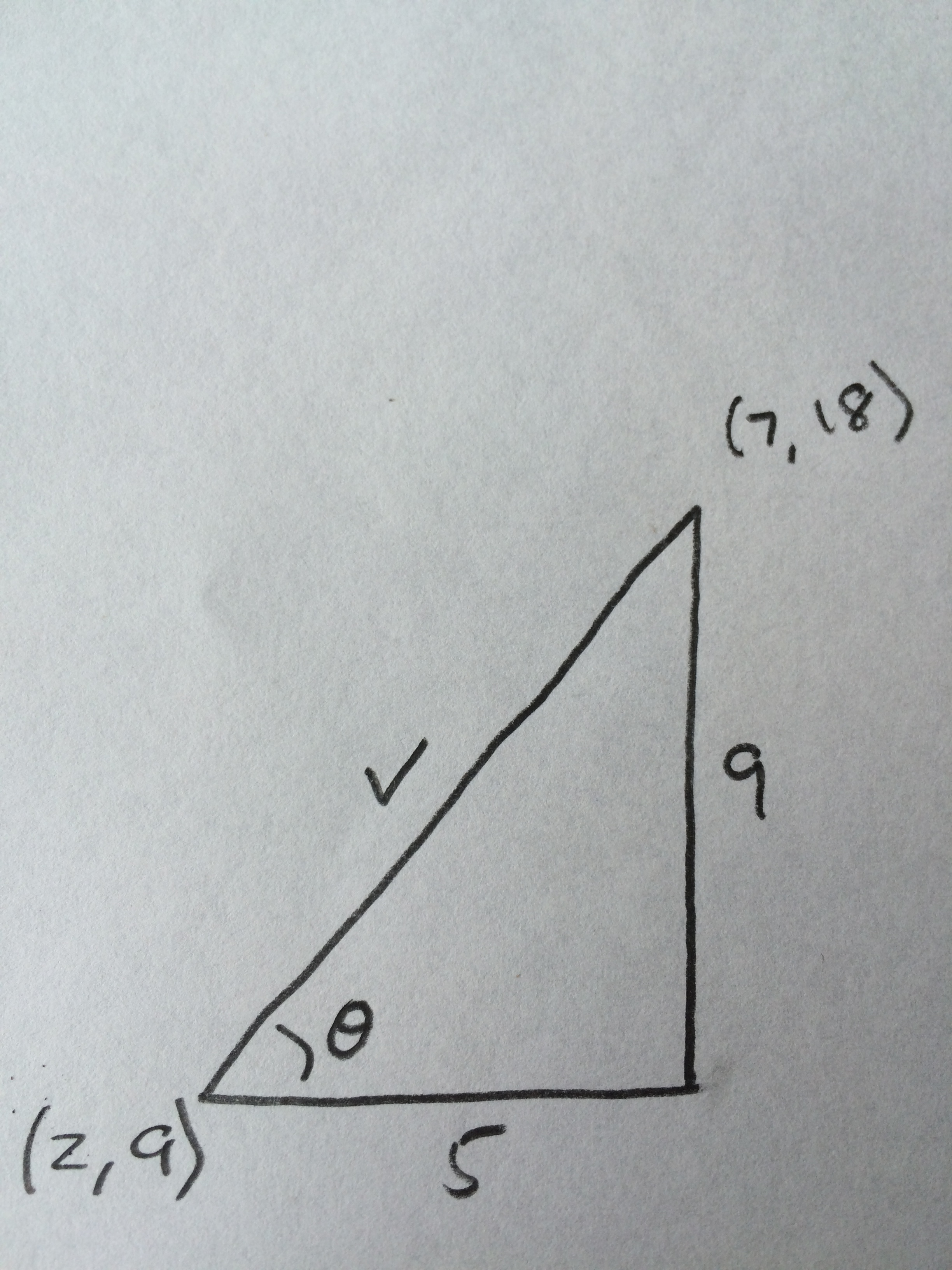At this point, we can essentially solve the problem as if we're finding the hypotenuse and angle of a right triangle.

Using the Pythagorean Theorem to find the length of the vector we get: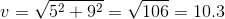Now we need to find the angle of the vector. We have all three sides of the triangle, so use the trig function that you are most comfortable with. The tangent function is used below because it uses sides that were given in the problem statement.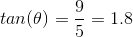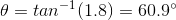### Example Question #2 : Find A Vector Equation When Given Two Points

Find the vector that starts at point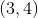and ends at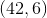and its magnitude.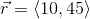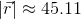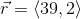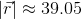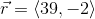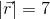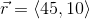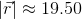Explanation:

To find the vector between two points, find the change between the points in theanddirections, or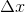and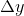. Then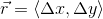. If it helps, draw a line from the starting point to the end point on a graph and look at the changes in each direction.

We see that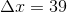and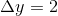, so our vector isTo find a vectors magnitude, we sum up the squares of each component and take the square root: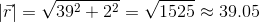### Example Question #3 : Find A Vector Equation When Given Two Points

Find the vector equation of the line through the points: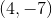and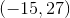.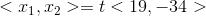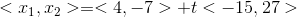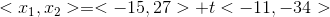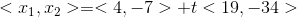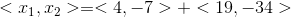Explanation:

The vector equation of the line through two points is the sum of one of the points and the direction vector between the two points scaled by a variable.

First we find the the direction vector by subtracting the two points: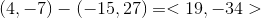.

Note that a line is continuous and defined on the real line. Then, we must scale the direction vector by a variable constant so as to define the line at each point. We then add one of the given points, so as to define the line through the given points. Either point can be chosen, but the correct answer uses the first point given.### Example Question #4 : Find A Vector Equation When Given Two Points

Given points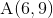and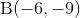, find a vector equation of the line passing these two points.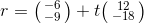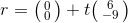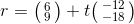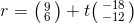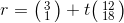Explanation:

Write the formula to find the vector equation of the line.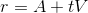Usingand, find the directional vectorby subtracting point A from B.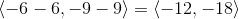Substitute the directional vectorand pointinto the formula.

A possible solution is:### All Precalculus Resources